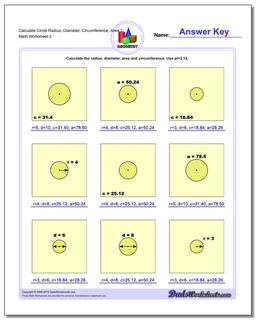# Math Worksheets: Basic Geometry: Basic Geometry: Calculate Circle Radius, Diameter, Circumference, Area 1 (Second Worksheet)## Calculate Circle Radius, Diameter, Circumference, Area 1 (Second Worksheet)

PropertyValue
DescriptionCalculate Circle Radius, Diameter, Circumference, Area 1: Calculate the radius, diameter, area and circumference given one circle fact. (Second Worksheet)
Resource TypeWorksheet